QID : 151 – How many numbers are there from 700 to 950 (including both) which are neither divisible by 3 nor by 7?

Options:
1) 107
2) 141
3) 144
4) 145

QID : 152 – A can complete a work in 20 days and B can complete the same work in 25 days. If both of them work together, then in 3 days what percent of the total work will be completed?

Options:
1) 9

2) 12

3) 25

4) 27

QID : 153 – The length of two parallel sides of a trapezium are 18 m and 24 m. If its height is 12 m, then what is the area (in m2) of the trapezium ?

Options:
1) 126
2) 252
3) 504
4) 1024

QID : 154 – If two successive discounts of 50% and 10% are offered, then what is the net discount (in %)?

Options:
1) 50
2) 55
3) 60
4) 65

QID : 155 – Three bottles of equal capacity containing mixture of milk and water in ratio 2 : 5, 3 : 4 and 4 : 5 respectively. These three bottles are emptied into a large bottle. What will be the ratio of milk and water respectively in the large bottle?

Options:
1) 73 : 106
2) 73 : 116
3) 73 : 113
4) 73 : 189

QID : 156 – The average age of 6 members of a family is 20 years. If the age of the servant is included, then the average age increase by 25%. What is the age (in years) of the servant?

Options:
1) 30

2) 35

3) 50

4) 55

QID : 157 – For an article the profit is 190% of the cost price. If the cost price increase by 10% but the selling price remains same, then profit is what percentage of selling price (approximately)?

Options:
1) 54
2) 62
3) 70
4) 163

## Study Kit for SSC CGL EXAM

QID : 158 – A, B and C are three students. A got 18% more marks than B and 12% less than C. If B got 220 marks, then how much marks C has got?

Options:
1) 230
2) 295
3) 240
4) 290

QID : 159 – Two people A and B are at a distance of 260 km from each other at 9:00 a.m. A immediately starts moving towards B at a speed of 25 km/h and at 11:00 a.m. B starts moving towards A at a speed of 10 km/hr. At what time (in p.m.) will they meet each other?

Options:
1) 5:00
2) 6:00
3) 6:30
4) 7:00

QID : 160 – If Rs 2500 becomes to Rs 2970.25 in 2 years at compound interest compounded annually, then what is the yearly rate of interest (in %)?

Options:
1) 7
2) 9
3) 11
4) 13

QID : 161 – If (1/x) + (1/y) + (1/z) = 0 and x + y + z = 9, then what is the value of x3 + y3 + z3 – 3xyz?

Options:
1) 81
2) 361
3) 729
4) 6561

QID : 162 – If x4 + (1/x4) = 34, then what is the value of x3 – (1/x3)?

Options:
1) 0
2) 6
3) 8
4) 14

QID : 163 – If x = 1 – y and x2 = 2 – y2, then what is the value of xy?

Options:
1) 1
2) 2
3) –1/2
4) –1

QID : 164 – If x + [1/(x + 7)] = 0, then what is the value of x – [1/(x + 7)]?

Options:
1) 3√5
2) 3√5 – 7
3) 3√5 + 7
4) 8

QID : 165 –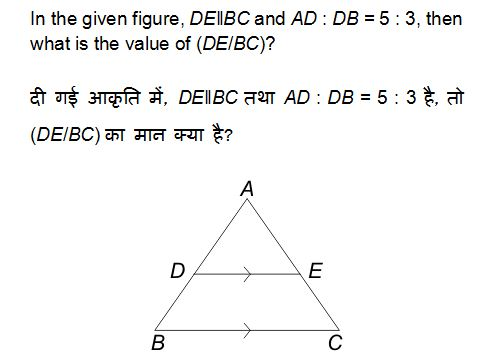Options:
1) 5/8

2) 2/3

3) 3/4

4) 5/3

QID : 166 – PQRS is a cyclic quadrilateral and PQ is the diameter of the circle. If ∠RPQ = 38°, then what is the value (in degrees) of ∠PSR?

Options:
1) 52
2) 77
3) 128
4) 142

QID : 167 – Smaller diagonal of a rhombus is equal to length of its sides. If length of each side is 6 cm, then what is the area (in cm2) of an equilateral triangle whose side is equal to the bigger diagonal of the rhombus?

Options:
1) 18√3

2) 27√3

3) 32√3

4) 36√3

QID : 168 –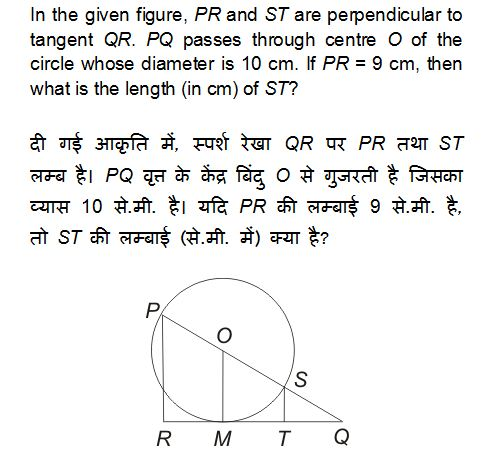Options:
1) 1

2) 1.25

3) 1.5

4) 2

QID : 169 – What is the simplified value of (sec A + cos A)(sec A – cos A)?

Options:
1) 2 tan2 A

2) 2 sin2  A

3) sin2 A tan2  A

4) sin2  A + tan2  A
Correct Answer: sin2 A + tan2  A

QID : 170 –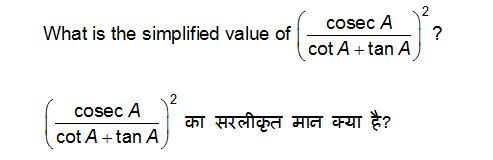Options:
1) 2cos2 A

2) 1 – sin2 A

3) sec2 A

4) secA tanA
Correct Answer: 1 – sin2 A

QID : 171 –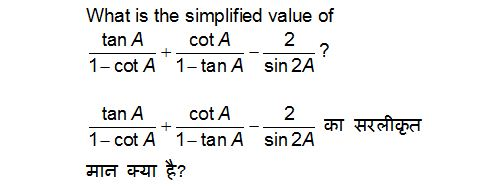Options:
1) –1

2) 0

3) 1

4) 2

QID : 172 –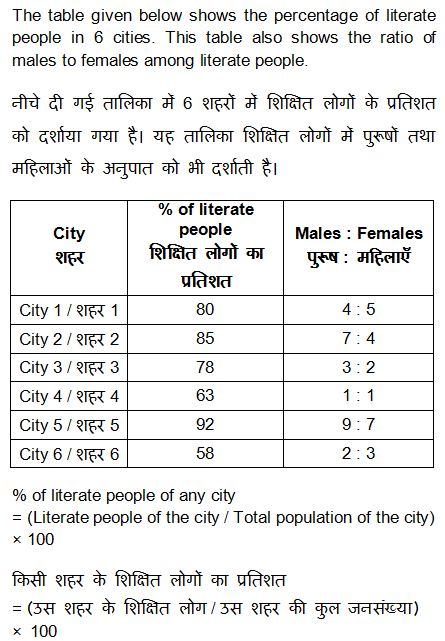If the total population of City 4 is 600000, then how many literate people are there in city 4?

Options:
1) 480000

2) 378000

3) 468000

4) 348000

QID : 173 –Total population of City 6 is 200000 and the total population of City 2 is 220000. What is the respective ratio of literate males of City 2 and literate females of City 6?

Options:
1) 348 : 595
2) 255 : 199
3) 595 : 348
4) 199 : 255

QID : 174 –If there are 259210 literate females in City 5, then what is the total population of City 5?

Options:
1) 644000

2) 354200

3) 690000

4) 483000